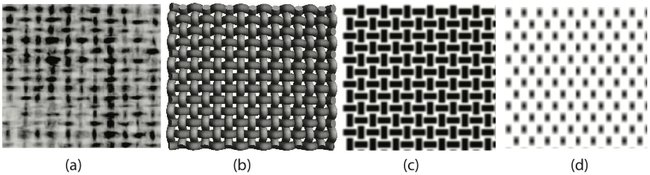### 5.1 Building a model

One of the hallmarks of a model-based approach to problem solving is to create a simplified schematic version of the problem, where the problem is easier to study and where candidate solutions can be attempted. After all, if a mathematical or computational technique cannot work reliably on a simplified model, there is little hope that it can solve the actual (more complex) problem. Several possible models for the thread counting problem are shown in figure . Image (a) shows a small portion of an X-ray image. Image (b) presents a physical model that attempts to imitate interwoven threads; this model attempts to convey the underlying three-dimensional physical structure of the canvas. While the eye may be familiar with such fake three-dimensionality, it does not appear to be particularly useful computationally. Model (c) presents a collection of rectangular shapes that crudely imitate the pattern of dark and light regions appearing in (a). Model (d) simplifies even further to a completely periodic set of sinusoidal undulations (which are discussed further below).1
A small portion of an X-ray image (a) and three simplified models. The model (b) mimics the three-dimensional undulations of the interwoven threads, model (c) abstracts this to a periodic pattern of rectangular shapes, and model (d) shows a product of two sinusoids, one in the horizontal and one in the vertical direction.

Counting the vertical (or horizontal) threads in figure 1(a) is analogous to estimating the number of columns (or rows) of rectangular patches in figure 1(c) or to estimating the number of black dots encountered in any given row or column of figure 1(d). There are a variety of algorithmic techniques that can be used to accomplish these tasks; some can be proven to be correct for the simple models, and demonstrated to be useful for the task. To see this distinction, assume (for the moment) that the image to be counted has the form of figure 1(d) Then the Fourier technique (described in the next section) is mathematically guaranteed to closely approximate the true number of dots per inch, which is the simple-model-surrogate that has been used for thread counts. But if the Fourier technique is applied to real X-ray images such as figure 1(a), there is no such guarantee. Instead, there is the empirical question: are the differences between (a) and (d) great enough to cause the solution to fail, or are the differences small enough that the method may be used?

Fortunately, the Fourier technique, when applied to canvas X-radiographs, usually provides answers that are very close to the actual thread counts. This is verified by comparing, in a large number of cases, manual thread counts to the automated counts. But it is always worthwhile to recognize that something may go wrong. The next section is intended to give an idea of how the Fourier method functions, so that such situations can be identified.